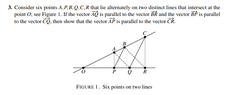# Proving parallel lines using points and vectors

• MHB
algebruh
Hey, this is a problem given to me by my prof for an assignment, and the TAs at my tutorials haven't been much help. Was wondering where to go with this question.

View attachment 9264

Also, I'm a uni freshman who isn't used to the whole concept of proofs, and a lot of what my profs say seem to be a slew of symbols and numbers before they even define anything, but I do the textbook readings and can comprehend those fairly easily. Was anyone on here's transition from high school math to university math a massive jump?

#### Attachments

•help.png
12.1 KB · Views: 35

skeeter
I'm going to assume you were exposed to the triangle proportionality theorem in a HS geometry course. To complete your proof, we require the use of its converse ... if you are not familiar or need a refresher, visit the attached link below.

For your problem, if we can show $\dfrac{OA}{AC} = \dfrac{OP}{PR}$, then by the converse of the triangle proportionality theorem $AP \parallel CR$.

Starting with $\Delta OCQ$, you are given $BP \parallel CQ$. Using the triangle proportionality theorem ...

$\dfrac{OB}{BC} = \dfrac{OP}{PQ} \implies \dfrac{OA+AB}{BC} = \dfrac{OP}{PQ} \implies \color{red}{(OA)(PQ)+(AB)(PQ)=(BC)(OP)}$

Same drill with $\Delta OBR$ ...

$\dfrac{OA}{AB} = \dfrac{OQ}{QR} \implies \dfrac{OA}{AB} = \dfrac{OP+PQ}{QR} \implies \color{red}{(OP)(AB)+(PQ)(AB)=(OA)(QR)}$

subtracting the second red equation from the first yields ...

$\color{red}(OA)(PQ)-(OP)(AB)=(BC)(OP)-(OA)(QR)$

rearranging ...

$\color{red} (OA)(PQ)+(OA)(QR) = (BC)(OP)+(OP)(AB)$

factoring both sides ...

$\color{red} (OA)(PQ+QR) = (OP)(AB+BC) \implies (OA)(PR) = (OP)(AC) \implies \dfrac{OA}{AC}= \dfrac{OP}{PR} \implies AP \parallel CR$